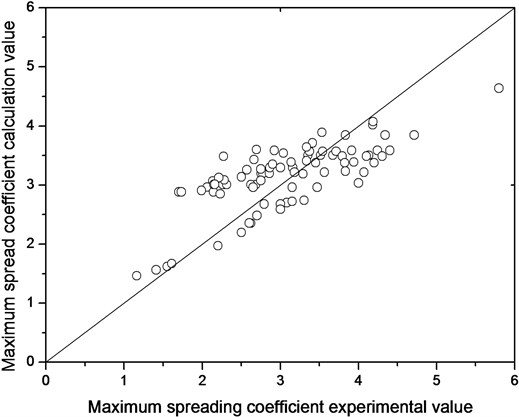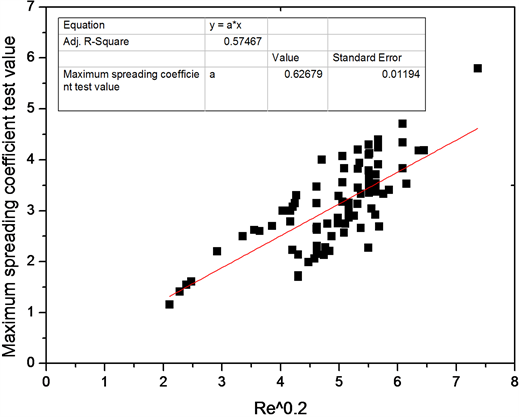# 液滴碰撞水平壁面的最大铺展系数The Maximum Spreading Factor of the Droplet Impacting the Horizontal Surface

DOI: 10.12677/IJM.2019.81001, PDF, HTML, XML, 下载: 422  浏览: 1,328

Abstract: There is plenty of semi-empirical formula or approximate formula on the maximum spreading factor of the droplet impacting the horizontal surface, but no theoretical solution now yet. The process of droplet impacting the surface is broken down into three sub-processes of drop, collision and spreading; the cylindrical droplet hypothesis is introduced, the laminar incompressible axisymmetric stagnation flow pattern is used, the theoretical formula of maximum spreading factor is deduced, and the theoretical formula combined with the literature data is verified. It shows that in the case where the Reynolds number is not more than 21,698, the theoretical formula and the actual result have a good compliance, and have a good explanation to some semi-empirical formula or approximate formula.

1. 引言Table 1. Semi-empirical Formula for Maximum Spread Coefficient

2. 假设模型

2.1. 无纲量参数

2.2. 跌落过程

$\begin{array}{c}{E}_{J0}={E}_{D0}+{E}_{Y0}+{E}_{S0}\\ =\frac{1}{2}m{U}_{0}^{2}+\frac{2m{\gamma }_{L}}{\rho {r}_{0}}+mg{r}_{0}\end{array}$ (1)

${E}_{\beta ,J0}=1+\frac{8}{We}+\frac{1}{Fr}$ (2)

$\frac{m{\gamma }_{L}}{\rho }\frac{1}{{R}_{1}}+\frac{1}{2}mg{H}_{1}=\frac{2m{\gamma }_{L}}{\rho }\frac{1}{{r}_{0}}+mg{r}_{0}$ (3)

${R}_{1}=\frac{1+\sqrt{\frac{8}{3}{\left(\frac{\rho g{r}_{0}^{2}}{{\gamma }_{L}}+1\right)}^{2}-\frac{5}{3}}}{4+\frac{2\rho g{r}_{0}^{2}}{{\gamma }_{L}}}{r}_{0}$ (4)

${\beta }_{1}=\frac{1+\sqrt{\frac{8}{3}{\left(Bd+1\right)}^{2}-\frac{5}{3}}}{4+2Bd}$ (5)

${r}_{0}\in \left(0,\infty \right)$ ，可得 ${\beta }_{1}\in \left(\frac{1}{2},\sqrt{\frac{2}{3}}\right)$

2.3. 碰撞过程

a) 在碰撞过程中液滴形状不发生变化；

b) 碰撞过程中动能守恒，液滴的垂直速度Uh转化为水平速度Ur

c) UH2等于零时碰撞结束。

$2\text{π}rh\text{d}r=-\text{π}{r}^{2}\text{d}h$ (6)

${U}_{H}=-\frac{2H}{R}{U}_{R}$ (7)

 (8)

$\left\{\begin{array}{l}{U}_{r}=ar\\ {U}_{h}=-2ah\end{array}$ (9)

${E}_{D2}=\frac{1}{4}m{U}_{R2}^{2}+\frac{1}{6}m{U}_{H2}^{2}=\frac{1}{2}m{U}_{0}^{2}$ (10)

2.4. 铺展过程

${E}_{\beta ,J3}={U}_{\beta }^{2}\left(\frac{1}{2}+\frac{64}{27{\beta }^{6}}\right)+\frac{4}{We\beta }+\frac{1}{6Fr{\beta }^{2}}$ (11)

3. 理论推导

$\left\{\begin{array}{l}{u}_{r}=r{f}^{\prime }\left(h\right)\\ {u}_{h}=-2f\left(h\right)\end{array}$ (12)

${\phi }^{‴}+2\phi {\phi }^{″}-{{\phi }^{\prime }}^{2}+1=0$ (13)

$\left\{\begin{array}{l}\xi =0;\text{\hspace{0.17em}}\phi =0,\text{\hspace{0.17em}}{\phi }^{\prime }=0\\ \xi =\infty ;\text{\hspace{0.17em}}{\phi }^{\prime }=1\end{array}$ (14)

${\delta }_{0.99}=\kappa \sqrt{\frac{\mu }{a\rho }}$ (15)

${E}_{\beta ,J0}-{E}_{\beta ,J3}={W}_{\beta ,N}+{W}_{\beta ,}{}_{S}$ (16)

3.1. 粘性耗散

$\varphi =2\mu \left[{\left(\frac{\partial {u}_{r}}{\partial r}\right)}^{2}+{\left(\frac{{u}_{r}}{r}\right)}^{2}+{\left(\frac{\partial {u}_{h}}{\partial h}\right)}^{2}\right]+\mu {\left(\frac{\partial {u}_{r}}{\partial h}\right)}^{2}$ (17)

$\varphi =12\mu {a}^{2}{{\phi }^{\prime }}^{2}+\rho {r}^{2}{a}^{3}{{\phi }^{″}}^{2}$ (18)

$\varphi \approx \rho {r}^{2}{a}^{3}{{\phi }^{″}}^{2}$ (19)

$\Phi ={\int }_{0}^{v}\varphi \text{d}V={\int }_{0}^{H}\varphi \text{π}{R}^{2}\text{d}h=\text{π}\sqrt{\rho \mu }{R}^{1.5}{U}_{R}^{2.5}{\int }_{0}^{\kappa }{{\phi }^{″}}^{2}\text{d}\xi$ (20)

$\epsilon ={\int }_{0}^{\kappa }{{\phi }^{″}}^{2}\text{d}\xi$ ，则

$\Phi =\text{π}\epsilon \sqrt{\rho \mu }{R}^{1.5}{U}_{R}^{2.5}$ (21)

$\begin{array}{c}{W}_{\beta }{}_{,N}=\frac{{\int }_{0}^{t}\Phi \text{d}t}{{E}_{D0}}=\frac{\pi \epsilon \sqrt{\rho \mu }}{{E}_{D0}}{\int }_{0}^{t}{R}^{1.5}{U}_{R}^{2.5}\text{d}t\\ =\frac{1}{\sqrt{Re}}\frac{3\sqrt{2}\epsilon }{2{r}_{0}^{2.5}{U}_{0}^{1.5}}{\int }_{{R}_{1}}^{R}{R}^{1.5}{U}_{R}^{1.5}\text{d}R\\ =\frac{3\sqrt{2}\epsilon }{2\sqrt{Re}}{\int }_{{\beta }_{1}}^{\beta }{\beta }^{1.5}{U}_{\beta }^{1.5}\text{d}\beta \end{array}$ (22)

3.2. 三相线耗散

$\tau \left(0\right)=\mu {\left(\frac{\partial {u}_{r}}{\partial h}\right)}_{h=0}={\phi }^{″}\left(0\right)\sqrt{\rho \mu }\frac{{U}_{R}^{1.5}}{{R}^{0.5}}$ (23)

$\text{d}{F}_{s}=2\text{πd}R\tau \left(0\right)=2\text{π}{\phi }^{″}\left(0\right)\sqrt{\rho \mu }\frac{{U}_{R}^{1.5}}{{R}^{0.5}}\text{d}R$ (24)

${W}_{\beta ,}{}_{s}=\frac{\int S\text{d}{F}_{s}}{{E}_{D0}}=\frac{2{\text{π}}^{2}{\phi }^{″}\left(0\right)\sqrt{\rho \mu }}{{E}_{D0}}{\int }_{{R}_{1}}^{R}{U}_{R}^{1.5}{R}^{1.5}\text{d}R=\frac{3\sqrt{2}\text{π}{\phi }^{″}\left(0\right)}{\sqrt{Re}}{\int }_{{\beta }_{1}}^{\beta }{\beta }^{1.5}{U}_{\beta }^{1.5}\text{d}\beta$ (25)

3.3. 铺展速度

$\omega =3\sqrt{2}\text{π}{\phi }^{″}\left(0\right)+\frac{3\sqrt{2}}{2}\epsilon =19.1804$

$1+\frac{8}{We}+\frac{1}{Fr}-\frac{1}{2}{U}_{\beta }^{2}=\frac{\omega }{\sqrt{Re}}{\int }_{{\beta }_{1}}^{\beta }{\beta }^{1.5}{U}_{\beta }^{1.5}\text{d}\beta$ (26)

$-{U}_{\beta }{{U}^{\prime }}_{\beta }=\frac{\omega }{\sqrt{Re}}{\beta }^{1.5}{U}_{\beta }^{1.5}$ (27)

${U}_{\beta }^{0.5}=C-\frac{\omega }{5\sqrt{Re}}{\beta }^{2.5}$ (28)

C为积分常数，将式(28)带入(26)可求得:

$C=\sqrt{2}{\left(1+\frac{8}{We}+\frac{1}{Fr}\right)}^{1/4}+\frac{\omega }{5\sqrt{Re}}{\beta }_{1}^{2.5}$ (29)

${U}_{\beta }^{0.5}=\sqrt{2}{\left(1+\frac{8}{We}+\frac{1}{Fr}\right)}^{1/4}-\frac{\omega }{5\sqrt{Re}}\left({\beta }^{2.5}-{\beta }_{1}^{2.5}\right)$ (30)

$\beta \propto {t}^{1/4}$ (31)

3.4. 最大铺展系数

${\beta }_{\mathrm{max}}^{2.5}=\frac{5\sqrt{2}}{\omega }\sqrt{Re}{\left(1+\frac{8}{We}+\frac{1}{Fr}\right)}^{1/4}+{\beta }_{1}^{2.5}$ (32)

${\beta }_{\mathrm{max}}\propto 0.626R{e}^{0.2}$ (33)

${\beta }_{\mathrm{max}}\propto 0.626R{e}^{0.2}{\left(1+8/We\right)}^{0.1}$ (34)

4. 数据验证

Table 2. Application and calculation data of maximum spreading coefficientFigure 1. Comparisons between experimental and calculated values of βmax

${\beta }_{\mathrm{max}}=0.627R{e}^{0.2}$ (35)Figure 2. Experimental Value of βmax and Re0.2 Fitting Figure

5. 结论

1) 建立液滴碰撞壁面的过程假设，利用轴对称驻地流动模型，得出最大铺展系数的理论关系式，该关系式有明确的物理意义，具有良好的解释力。

2) 通过实验数据的对比，计算值和实验值有较强的符合性。

  Chandra, S. and Avedisian, C.T. (1991) On the Collision of a Droplet with a Solid Surface. Proceedings: Mathematical and Physical Sciences, 432, 13-41.  Scheller, B.L. and Bousfield, D.W. (1995) Newtonian Drop Impact with a Solid Surface. AIChE Journal, 41, 1357-1367.  Pasandideh-Fard, M., Qiao, Y.M., Chandra, S. and Mostaghimi, J. (1996) Capillary Effects during Droplet Impact on a Solid Surface. Physics of Fluids, 8, 650-659. https://doi.org/10.1063/1.868850  Kwon, T.J. (2003) Simulating Collisions of Droplets with Walls and Films Using a Level Set Method. Purdue University, Purdue.  Clanet, C., Béguin, C., Richard, D. and Quéré, D. (2004) Maximal Deformation of an Impacting Drop. Journal of Fluid Mechanics, 517, 199-208.  Ukiwe, C. and Kwok, D.Y. (2005) On the Maximum Spreading Diamater of Impacting Droplets on Well-Prepared Solid Surfaces. Langmuir, 21, 666-673. https://doi.org/10.1021/la0481288  Roisman, I.V. (2009) Inertia Dominated Drop Collisions. II. An Analytical Solution of the Navier-Stokes Equations for a Spreading Viscous Film. Physics of Fluids, 21, 052104. https://doi.org/10.1063/1.3129283  Eggers, J., Fontelos, M., Josserand, C. and Zaleski, S. (2010) Drop Dynamics after Impact on a Solid Wall: Theory and Simulations. Physics of Fluids, 22, 062101. https://doi.org/10.1063/1.3432498  陈石, 王辉, 沈胜强, 梁刚涛. 液滴振荡模型及与数值模拟的对比[J]. 物理学报, 2013, 62(20): 204702.  李大树. 液滴撞壁动力学及传热机制研究[D]: [博士学位论文]. 北京: 中国石油大学, 2015.  Lee, J.B., Laan, N., de Bruin, K.G., Skantzaris, G., et al. (2016) Universal Rescaling of Drop Impact on Smooth and Rough Surfaces. Journal of Fluid Mechanics, 786, R4.  Huang, H.M. and Chen, X.P. (2018) Energetic Analysis of Drop’s Maximum Spreading on Solid Surface with Low Impact Speed. Physics of Fluids, 30, 022106. https://doi.org/10.1063/1.5006439  温原. 液滴铺展过程的能量转换[J]. 力学研究, 2018, 7(4): 121-125.  Hiemenz, K. (1911) Die Grenzschicht an einem in den gleichformigen Flussigkeitsstrom eingetauchten garaden Kreiszylinder. Dinglers Polytech. J, 326, 321.  朱克勤, 徐春晓. 粘性流体力学[M]. 北京: 高等教育出版社, 2009.  Frössling, N. (1940) Verdunstung, Wärmeübertragung und Geschwindigkeitverteilung bei Zwei-dimensionaler und Rotationssy mmetrischen Laminarer Grenzschichtstromung.  袁泉子, 沈文豪, 赵亚溥. 移动接触线的物理力学研究[J]. 力学进展, 46(1): 343-381.  H.史里希廷. 边界层理论[M]. 北京: 科学出版社, 2017.  周龙玉. 液滴碰撞试验与数值研究[D]: [硕士学位论文]. 哈尔滨: 哈尔滨工程大学, 2013.  毕菲菲, 郭亚丽, 沈胜强, 等. 液滴撞击固体表面铺展特性的实验研究[J]. 物理学报, 2012, 61(18): 295-300.  杨宝海, 朱恂, 王宏, 等. 不同直径液滴撞击亲水壁面动态特性实验研究[J]. 工程热物理学报, 2014, 35(1): 91-94.  张帆, 陈凤, 薄涵亮. 不同亲疏水表面液滴动力学行为实验研究[J]. 原子能科学技术, 2015, 49(1): 288-293.  崔洁, 陆军军, 陈雪莉. 液滴高速撞击固体板面过程的研究[J]. 化学反应工程与工艺, 2008, 24(5): 390-394.  谢亚星, 慕松, 陈星名. 液滴撞击枸杞叶片铺展特性实验研究与仿真分析[J]. 中国农机化学报, 2017, 38(9): 70-74.  郭亚丽, 陈桂影, 沈胜强, 等. 盐水液滴撞击固体壁面接触特性实验研究[J]. 工程热物理学报, 2015, 36(7): 1547-1552.  李长宁, 夏振炎, 李建军, 等. 液滴撞击有机玻璃固壁的实验和数值研究[J]. 工程塑料应用, 2014, 42(3): 39-43.  Ford, R.E. and Furmidge, C.G.L. (1967) Impact and Spreading of Spray Drops on Foliar Surfaces. Society of Chemical Industry, London, 417-432.  Chandra, S. and Avedisian, C.T. (1991) On the Collision of a Droplet with a Solid Surface. Proceedings of the Royal Society A, 432, 13. https://doi.org/10.1098/rspa.1991.0002  Bhola, R. and Chandra, S. (1995) Freezing of Droplets Colliding with a Cold Surface. ASME HTD, Vol. 306, 181.  Pasandideh Fard, M., Qiao, Y.M. and Chandra, S. (1996) Capillary Effects during Droplet Impact on a Solid Surface. Physics of Fluids, 8, 650-659. https://doi.org/10.1063/1.868850